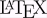Clearly it would be nice to be able to enter equations and the like, but there's only so much you can do in HTML. It's suggested that a limited form of maths mode would be useful.

HTML has some pretty fundamental limitations when it comes to type-setting. Originally it was specifically not intended to allow tight control of page-layout - it was as its name says: Hyper Text Markup Language. "Hyper Text" because it allows links, and "Markup Language" because it allows the text to be marked up with bold, italic, and other simple changes.

When it hit the mass market, however, people wanted tighter control over how their pages looked. HTML has accordingly been enhanced with tables and other layout features, but it still lacks basic mathematics layout. This is, to be fair, because maths layout is hard. Really hard. There is an entire system called(pronounced lay-tek) designed to deal with it.

Having said all that, we've now provided a facility for creating equations. You have to type the equation into the text box - you can't cut-n-paste pretty pictures as you can in MathType and similar packages, but you can get some effective equations. Just type EQN : and then your equation.

Here are some really simple equations to give you the idea:
 This text in the edit box produces this image EQN : x^2 $x^2$ EQN : x_0 $x_0$ EQN : \frac{num}{den} $\frac{num}{den}$ EQN : \int{x}.dx $\int{x}.dx$ EQN : x^2+y^2=z^2 $x^2+y^2=z^2$

Now for some more complex examples:
 This text in the edit box produces this image EQN : \int_{-\infty}^{\infty}{x^2}dx $\int_{-\infty}^{\infty}{x^2}dx$ EQN : x\longrightarrow^gy $x\longrightarrow^gy$ EQN : y=\left\{\text{this\\that}\right. $y=\left\{\text{this\\that}\right.$ EQN : \begin{matrix}a_1&a_2&a_3\\b_1&b_2&b_3\end{matrix} $\begin{matrix}a_1&a_2&a_3\\b_1&b_2&b_3\end{matrix}$ EQN : \normalsize{f}(x)=\int\limits_{-\infty}^xe^{-t^2}dt $\normalsize{f}(x)=\int\limits_{-\infty}^xe^{-t^2}dt$ EQN : \LARGE{x}=\frac{-b\pm\sqrt{b^2-4ac}}{2a} $\LARGE{x}=\frac{-b\pm\sqrt{b^2-4ac}}{2a}$ EQN : \int_0^\infty{x^2+y_0}(\frac{a+b}{c})dx $\int_0^\infty{x^2+y_0}(\frac{a+b}{c})dx$

The "limits" keyword can be used to put things above or below the previous symbol:

• EQN : x\limits^{above}_{below} - $x\limits{-}{.}$
That's not required for some symbols: $\hat{x}$

Bold is achieved using \bf - limit its scope by using braces:

• ${\bf~x}y$
In the last two examples note the use of a tilde ("~") where we would normally want a space.